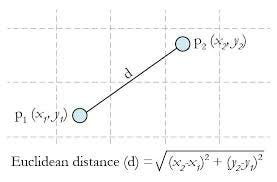Euclidean Distance

# Object Tracking — Referenced with the ’n’ previous frame using Euclidean distance.

Before you will check this blog when you are going to read this blog. Because I have explained how to track the object using Euclidean distance by referring to the previous frame.

In this blog explains how to track each object by referring to the ’n’ previous frame. The ’n’ means the number of frames to refer. Now I would take 8 frames to refer.

I have explained to the detection and tracking techniques in my previous blog which is I mentioned above.

#### Concept:

Only save the 8 frames of middle points of each bounding box and corresponding labels to find the distance between the current frame and the previous 8 frames.

#### Frames Values:

ref-8-frames-points = [[(208, 268)], [(214, 271)], [(226, 273)], [(230, 271)], [(628, 300)], [(619, 301), (249, 257)], [(250, 258), (606, 274)], [(250, 271), (602, 256)]]
ref-8-frames-lables = [, , , , , [3, 2], [2, 3], [2, 3]]

#### Frames Values Flatten:

ref-8-frames-points-flatten = [(208, 268), (214, 271), (226, 273), (230, 271), (628, 300), (619, 301), (249, 257), (250, 258), (606, 274), (250, 271), (602, 256)]
ref-8-frames-lables-flatten = [2, 2, 2, 2, 3, 3, 2, 2, 3, 2, 3]

#### Explanation:

Line 209–211: Totally 8 frames values to store. Once the length is filled and deleted the first frame value. It’s a queue concept.
Line 212–215: Save all frames and reshape into 2 dimensional.

#### Pros:

1. I have saved the previous n frames points. If the model does not detect one or less than n, it is not a problem.

#### Cons:

1. If the two persons are crossing, maybe interchange their unique id.
2. If the model does not detect n frame, then the unique label changed.\